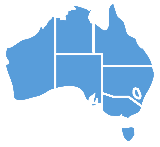Keywords: total, sum, add up

## Sign Definition

### As a Noun

1. The number you get when you add several numbers together. English = total.
2. An amount of money. English = sum.
3. A simple calculation in arithmetic. English = sum.

### As a Verb or Adjective

1. To add several numbers together. English = total, add up.
2. To add several amounts of money together. English = sum.